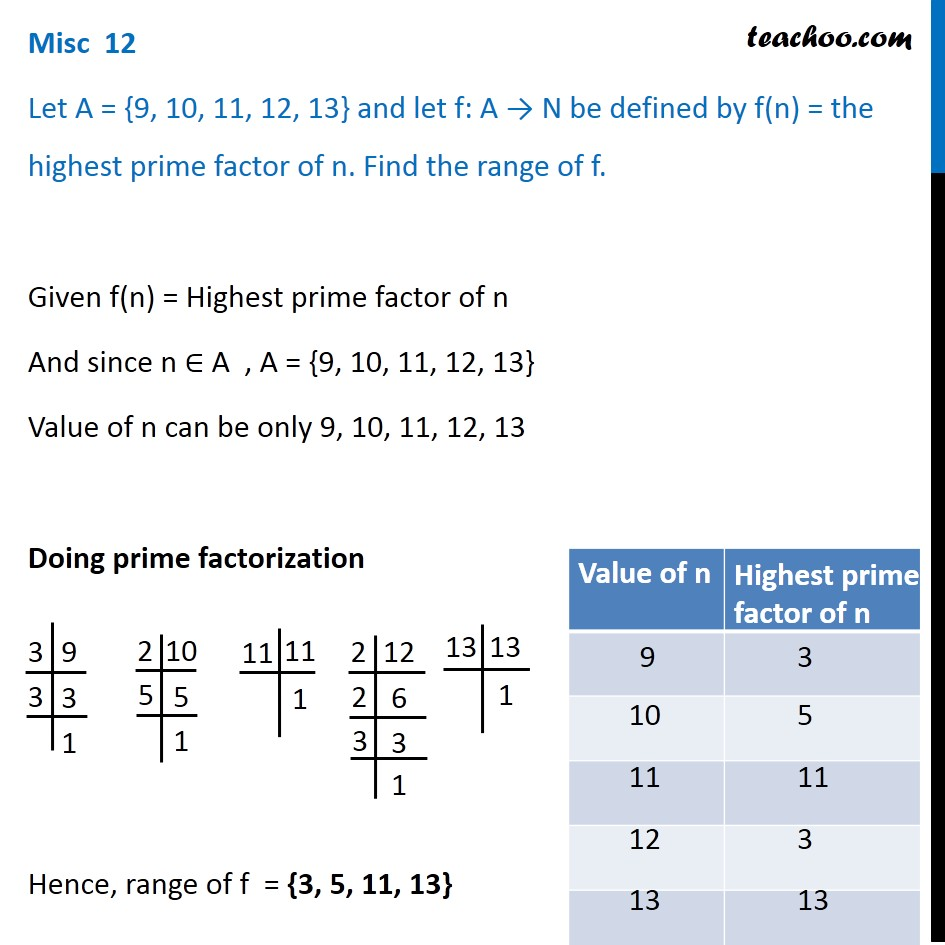Finding Domain and Range - General Method

Chapter 2 Class 11 Relations and Functions
Concept wiseLearn in your speed, with individual attention - Teachoo Maths 1-on-1 Class

### Transcript

Misc 12 Let A = {9, 10, 11, 12, 13} and let f: A → N be defined by f(n) = the highest prime factor of n. Find the range of f. Given f(n) = Highest prime factor of n And since n ∈ A , A = {9, 10, 11, 12, 13} Value of n can be only 9, 10, 11, 12, 13 Doing prime factorization Hence, range of f = {3, 5, 11, 13}